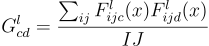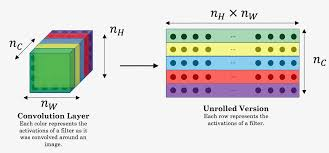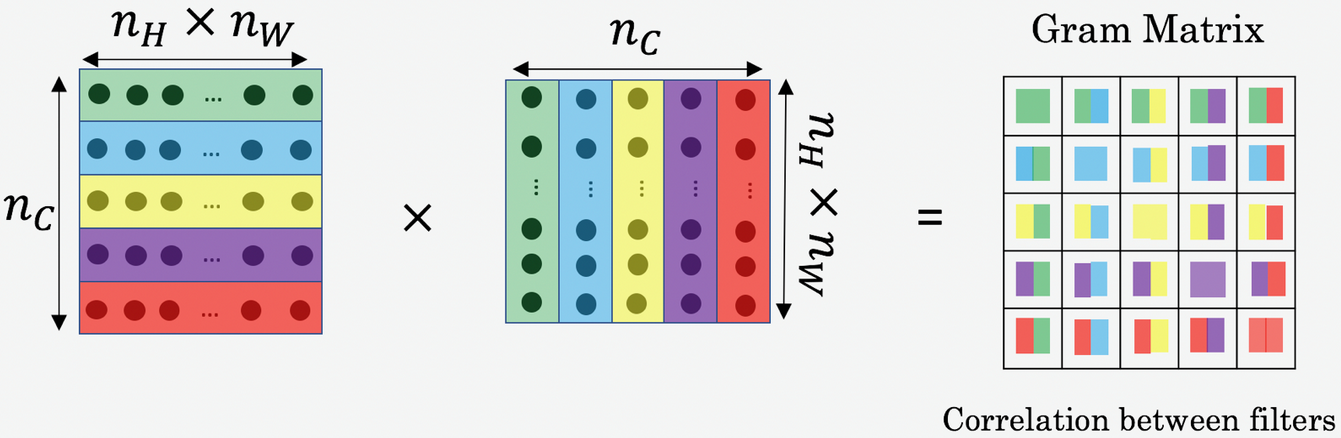# Calculating Style

The content of an image is represented by the values of the intermediate feature maps.

On the other hand, the style of an image can be described by the means and correlations across the different feature maps. We calculate a Gram matrix that includes this information by taking the outer product of the feature vector with itself at each location and averaging that outer product over all locations. This Gram matrix can be calculated for a particular layer as:This can be implemented concisely using the `tf.linalg.einsum` function:

We shall now define a function to calculate the gram matrix, given the style activations from a layer.

INSTRUCTIONS
• Define the function `gram_matrix` and pass the `input_tensor`, which would potentially be the output activations from an intermediate style layer of the input image. We shall implement the following steps in the function:

• Calculate the numerator of the above-mentioned gram matrix formula.

• Divide the thus obtained result with the multiplication of the width and height of the image.

Use the below code to calculate the gram matrix of given input tensor:

``````def gram_matrix(input_tensor):

result = tf.linalg.einsum('bijc,bijd->bcd', input_tensor, input_tensor)

input_shape = tf.shape(input_tensor)
num_locations = tf.cast(input_shape*input_shape, tf.float32)

return result/(num_locations)
``````
• -

No hints are availble for this assesment

Answer is not availble for this assesment

Note - Having trouble with the assessment engine? Follow the steps listed here